# How to Split Number into Different Columns in Excel

Sometimes we get a number consists of some parts, for example, an ID for a student 100100120050121, the first 4 digits 1001 is class ID, next 001 is student ID, the last part 20050121 is the student’s birthday. If we want to split this number into three columns refer to above three parts, how can we do? Actually, in excel, we can use Text to Columns function to split number, sentence or string into different columns properly. This article will show you the details of split a number step by step.

For example: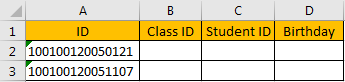Refer to above rule, we need to split number in column A to column B, C, D.

## Split Number into Different Columns in Excel

Step 1: Copy A2 to B2 firstly.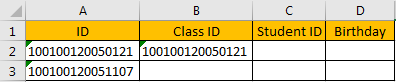Step 2: Select B2, then click Date in ribbon, click Text to Columns in Data Tools group.Verify that ‘Convert Text to Columns Wizard’ window pops up. Total 3 steps.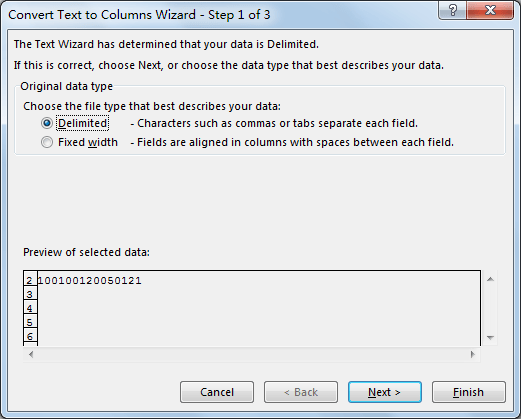Step 3: Check on ‘Fixed width’, and click Next. As we want to split a number, so we can split it by different width; if there are some characters exist in number, you can keep default option ‘Delimited’, and set different character per your demands as separator.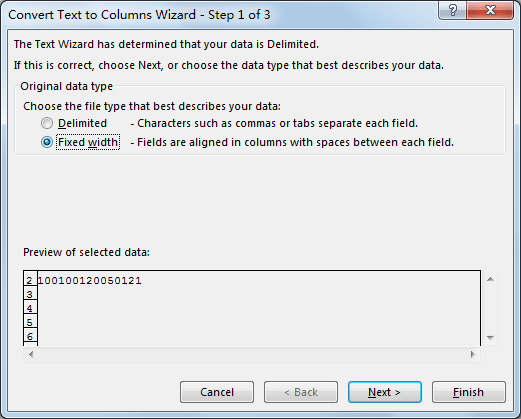Step 4: Add two break lines to split number refer to description. Verify that number is split by break lines properly in Data preview field. Then click Next.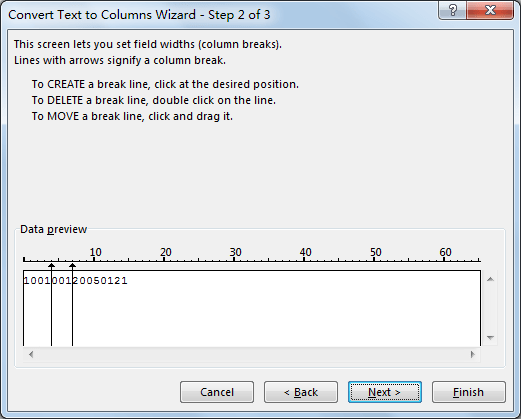Step 5: In the last step, select the format for each section. We select Text in this case. Then click Finish.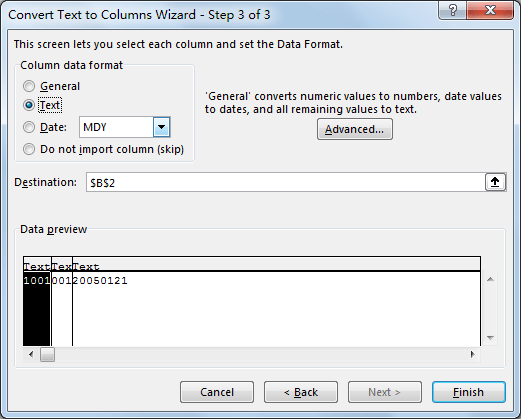Step 6: Verify that number is split properly.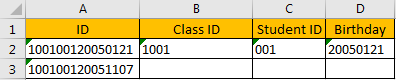Comment:

If number is displayed as ‘1001 001 20050121’ with two space to connect each part, you can check on ‘Delimited’ in ‘Convert Text to Columns Wizard’ step 2 and check on ‘Space’ as separator to split number. See screenshot below: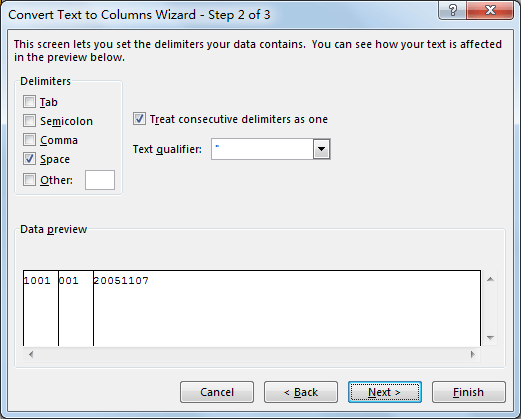Related Posts

If Cell is This Value or That Value

IF function is frequently used in Excel worksheet to return you expect “true value” or “false value” based on the result of logical test. If you want to see if a cell is A or B, and if one of ...

If Value is Greater Than A Certain Value

IF function is frequently used in Excel worksheet to return you expect “true value” or “false value” based on the logical test result. If you want to see if a value in one cell is greater than a specific value, ...

If Cell is Not Blank

IF function is frequently used in Excel worksheet to return you expect “true value” or “false value” based on the result of created logical test. If you want to see if a cell is blank or not, and leave some ...

VBA Macro For VLOOKUP From Another Sheet

In the previous post, you should know that how to fix or remove the #N/A error when using VLOOKUP formula to lookup value from another sheet. And this post will show you how to use VBA code to vlookup data ...

If Cell is Blank

IF function is frequently used in Excel worksheet to return you expect “true value” or “false value” based on the result of created logical test. If you want to see if a cell is blank or not, and leave some ...

If Cell Equals Certain Text String

IF function is frequently used in Excel worksheet to return you expect “true value” or “false value” based on the result of created logical test. If you want to see if cell equals a certain text string like “Win”, you ...

If Cell Contains Either Text1 or Text2

IF function is frequently used in Excel worksheet to return “true value” or “false value” based on the logical test result. If you want to see if cell contains certain substring1 like “abc” or substring2 like “def”, and returns true ...

If Cell Contains Certain Text OR Equals Certain Text

IF cell equals certain text IF function is frequently used in Excel worksheet to return “true value” or “false value” based on the logical test result. If you want to test values to see if they equal certain text like ...

VLOOKUP From Another Sheet Not Working

In the previous post, you should know that how to fix or remove the #N/A error when using VLOOKUP formula to lookup value from another sheet. And this post will show you reasons why your VLOOKUP formula is not working ...

If Cell Begins with One of Three Supplied Characters

If you want to test values to see if they begin with some given specific characters like “x”, ”y”, or “z”, you can create a formula with COUNTIF and SUM functions to return results. EXAMPLE You can see “TRUE” or ...

Sidebar